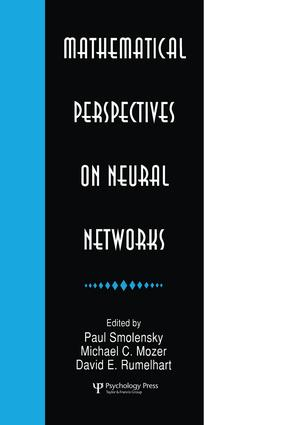# Mathematical Perspectives on Neural Networks

## 1st Edition

Psychology Press

##### Purchasing Options:\$ = USD
Paperback: 9781138876293
pub: 2015-05-07
SAVE ~\$11.99
\$59.95
\$47.96
x
Hardback: 9780805812015
pub: 1996-06-01
SAVE ~\$57.00
\$285.00
\$228.00
x
eBook (VitalSource) : 9780203772966
pub: 2013-05-13
from \$28.98

FREE Standard Shipping!

### Description

Recent years have seen an explosion of new mathematical results on learning and processing in neural networks. This body of results rests on a breadth of mathematical background which even few specialists possess. In a format intermediate between a textbook and a collection of research articles, this book has been assembled to present a sample of these results, and to fill in the necessary background, in such areas as computability theory, computational complexity theory, the theory of analog computation, stochastic processes, dynamical systems, control theory, time-series analysis, Bayesian analysis, regularization theory, information theory, computational learning theory, and mathematical statistics.

Mathematical models of neural networks display an amazing richness and diversity. Neural networks can be formally modeled as computational systems, as physical or dynamical systems, and as statistical analyzers. Within each of these three broad perspectives, there are a number of particular approaches. For each of 16 particular mathematical perspectives on neural networks, the contributing authors provide introductions to the background mathematics, and address questions such as:

* Exactly what mathematical systems are used to model neural networks from the given perspective?

* What formal questions about neural networks can then be addressed?

* What are typical results that can be obtained? and

* What are the outstanding open problems?

A distinctive feature of this volume is that for each perspective presented in one of the contributed chapters, the first editor has provided a moderately detailed summary of the formal results and the requisite mathematical concepts. These summaries are presented in four chapters that tie together the 16 contributed chapters: three develop a coherent view of the three general perspectives -- computational, dynamical, and statistical; the other assembles these three perspectives into a unified overview of the neural networks field.

### Reviews

Although the material is advanced and technical, the volume has a "multilayer" structure including a general overview, and there are overviews of each of the main parts (including summary tables of key results). These surveys, all written by Smolensky (John Hopkins University), provide an excellent introduction to the papers and their context, making the material accessible to upper-division undergraduates, graduate students, or faculty.

CHOICE

### Table of Contents

Contents: Preface: Multilayer Structure of the Book and Its Summaries. P. Smolensky, Overview: Computational, Dynamical, and Statistical Perspectives on the Processing and Learning Problems in Neural Network Theory. Part I: Computational Perspectives. P. Smolensky, Overview: Computational Perspectives on Neural Networks. S. Franklin, M. Garzon, Computation by Discrete Neural Nets. I. Parberry, Circuit Complexity and Feedforward Neural Networks. J.S. Judd, Complexity of Learning. E.H.L Aarts, J.H.M. Korst, P.J. Zwietering, Deterministic and Randomized Local Search. M.B. Pour-El, The Mathematical Theory of the Analog Computer. Part II: Dynamical Perspectives. P. Smolensky, Overview: Dynamical Perspectives on Neural Networks. M.W. Hirsch, Dynamical Systems. L.F. Abbott, Statistical Analysis of Neural Networks. K.S. Narendra, S-M. Li, Neural Networks in Control Systems. A.S. Weigend, Time Series Analysis and Prediction. Part III: Statistical Perspectives. P. Smolensky, Overview: Statistical Perspectives on Neural Networks. R. Szeliski, Regularization in Neural Nets. D.E. Rumelhart, R. Durbin, R. Goldin, Y. Chauvin, Backpropagation: The Basic Theory. J. Rissanen, Information Theory and Neural Nets. A. Nádas, R.L. Mercer, Hidden Markov Models and Some Connections with Artificial Neural Nets. D. Haussler, Probably Approximately Correct Learning and Decision-Theoretic Generalizations. H. White, Parametric Statistical Estimation with Artificial Neural Networks. V.N. Vapnik, Inductive Principles of Statistics and Learning Theory.

### Subject Categories

##### BISAC Subject Codes/Headings:
PSY008000
PSYCHOLOGY / Cognitive Psychology